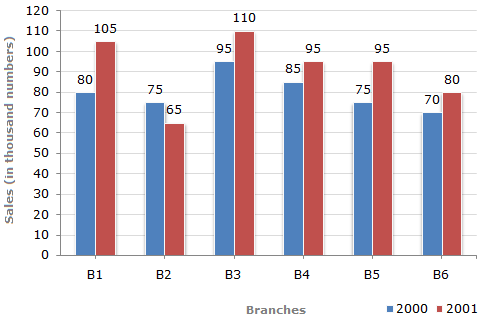# Data Interpretation - Bar Charts

## Why Data Interpretation Bar Charts?

In this section you can learn and practice Data Interpretation Questions based on "Bar Charts" and improve your skills in order to face the interview, competitive examination and various entrance test (CAT, GATE, GRE, MAT, Bank Exam, Railway Exam etc.) with full confidence.

## Where can I get Data Interpretation Bar Charts questions and answers with explanation?

IndiaBIX provides you lots of fully solved Data Interpretation (Bar Charts) questions and answers with Explanation. Solved examples with detailed answer description, explanation are given and it would be easy to understand. All students, freshers can download Data Interpretation Bar Charts quiz questions with answers as PDF files and eBooks.

## Where can I get Data Interpretation Bar Charts Interview Questions and Answers (objective type, multiple choice)?

Here you can find objective type Data Interpretation Bar Charts questions and answers for interview and entrance examination. Multiple choice and true or false type questions are also provided.

## How to solve Data Interpretation Bar Charts problems?

You can easily solve all kind of Data Interpretation questions based on Bar Charts by practicing the objective type exercises given below, also get shortcut methods to solve Data Interpretation Bar Charts problems.

The bar graph given below shows the sales of books (in thousand number) from six branches of a publishing company during two consecutive years 2000 and 2001.

Sales of Books (in thousand numbers) from Six Branches - B1, B2, B3, B4, B5 and B6 of a publishing Company in 2000 and 2001.1.

What is the ratio of the total sales of branch B2 for both years to the total sales of branch B4 for both years?

 A. 2:3 B. 3:5 C. 4:5 D. 7:9

Answer: Option D

Explanation:

 Required ratio = (75 + 65) = 140 = 7 . (85 + 95) 180 9

2.

Total sales of branch B6 for both the years is what percent of the total sales of branches B3 for both the years?

 A. 68.54% B. 71.11% C. 73.17% D. 75.55%

Answer: Option C

Explanation:

Required percentage
 =(70 + 80) x 100% (95 + 110)
 =150 x 100% 205
= 73.17%.

3.

What percent of the average sales of branches B1, B2 and B3 in 2001 is the average sales of branches B1, B3 and B6 in 2000?

 A. 75% B. 77.5% C. 82.5% D. 87.5%

Answer: Option D

Explanation:

Average sales (in thousand number) of branches B1, B3 and B6 in 2000

 = 1 x (80 + 95 + 70) =245. 3 3

Average sales (in thousand number) of branches B1, B2 and B3 in 2001

 = 1 x (105 + 65 + 110) =280. 3 3Required percentage =245/3 x 100% =245 x 100% = 87.5%. 280/3 280

4.

What is the average sales of all the branches (in thousand numbers) for the year 2000?

 A. 73 B. 80 C. 83 D. 88

Answer: Option B

Explanation:

Average sales of all the six branches (in thousand numbers) for the year 2000

 = 1 x [80 + 75 + 95 + 85 + 75 + 70] 6

= 80.

5.

Total sales of branches B1, B3 and B5 together for both the years (in thousand numbers) is?

 A. 250 B. 310 C. 435 D. 560

Answer: Option D

Explanation:

Total sales of branches B1, B3 and B5 for both the years (in thousand numbers)

= (80 + 105) + (95 + 110) + (75 + 95)

= 560.

#### Current Affairs 2019

Interview Questions and Answers